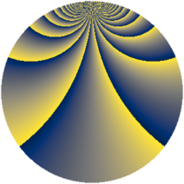# Properties

 Label 2415.2.cpLevel $2415$ Weight $2$ Character orbit 2415.cp Rep. character $\chi_{2415}(83,\cdot)$ Character field $\Q(\zeta_{44})$ Dimension $7520$ Sturm bound $768$

# Related objects

## Defining parameters

 Level: $$N$$ $$=$$ $$2415 = 3 \cdot 5 \cdot 7 \cdot 23$$ Weight: $$k$$ $$=$$ $$2$$ Character orbit: $$[\chi]$$ $$=$$ 2415.cp (of order $$44$$ and degree $$20$$) Character conductor: $$\operatorname{cond}(\chi)$$ $$=$$ $$2415$$ Character field: $$\Q(\zeta_{44})$$ Sturm bound: $$768$$

## Dimensions

The following table gives the dimensions of various subspaces of $$M_{2}(2415, [\chi])$$.

Total New Old
Modular forms 7840 7840 0
Cusp forms 7520 7520 0
Eisenstein series 320 320 0

## Trace form

 $$7520q - 44q^{7} + O(q^{10})$$ $$7520q - 44q^{7} - 44q^{15} + 560q^{16} + 52q^{18} - 44q^{21} - 88q^{25} - 44q^{28} - 44q^{30} - 88q^{36} - 22q^{42} - 88q^{43} - 208q^{46} - 88q^{51} - 44q^{57} + 24q^{58} - 44q^{60} - 22q^{63} - 88q^{67} + 32q^{70} + 28q^{72} - 28q^{78} - 184q^{81} - 40q^{85} - 440q^{88} - 256q^{93} + O(q^{100})$$

## Decomposition of $$S_{2}^{\mathrm{new}}(2415, [\chi])$$ into newform subspaces

The newforms in this space have not yet been added to the LMFDB.